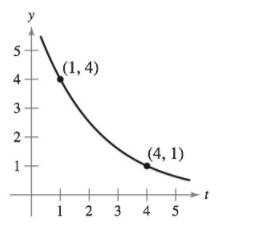Chapter 6, Problem 28RE

Chapter
Section
Textbook Problem

Finding an Exponential Function In Exercises 25-28, find the exponential function y = C e k t that passes through the two given points.To determine

To calculate: Theexponential function y=Cekt that passes through the points (1,4) and (4,1).

Explanation

Given:

The graph of y=Cekt is:

Formula used:

lnex=x

Calculation:

Now, put the value of t1 and y1 from the point (1,4) in y=Cekt:

4=Cek4=CekC=4ek

Put t2=4,y2=1 from point (4,1) in y=Cekt

1=Ce4k1=Ce4kC=e4k

Still sussing out bartleby?

Check out a sample textbook solution.

See a sample solution

The Solution to Your Study Problems

Bartleby provides explanations to thousands of textbook problems written by our experts, many with advanced degrees!

Get Started

Convert the expressions in Exercises 6584 to power form. 4x23+x3/2623x2

Finite Mathematics and Applied Calculus (MindTap Course List)

In Exercises 49-62, find the indicated limit, if it exists. 50. limx2x24x+2

Applied Calculus for the Managerial, Life, and Social Sciences: A Brief Approach

In Problems 19-44, factor completely. 21.

Mathematical Applications for the Management, Life, and Social Sciences